U.S. Department of Transportation
1200 New Jersey Avenue, SE
Washington, DC 20590
202-366-4000

Federal Highway Administration Research and Technology
Coordinating, Developing, and Delivering Highway Transportation InnovationsThis report is an archived publication and may contain dated technical, contact, and link information
 Federal Highway Administration > Publications > Research Publications > 08019 > Development of a Driver Vehicle Module (DVM) for the Interactive Highway Safety Design Model (IHSDM)
Publication Number: FHWA-HRT-08-019
Date: November 2007

# Development of a Driver Vehicle Module (DVM) for the Interactive Highway Safety Design Model (IHSDM)

PDF files can be viewed with the Acrobat® Reader®

# SECTION 3. SPECIFICATION OF THE DVM

## Introduction

This section provides a systematic delineation of the DVM components. Each of the major DVM components are discussed in terms of purpose, assumptions and limitations, computations, and additional specifications, as defined here:

• Purpose: A short summary of the role of this component in the DVM.
• Assumptions and Limitations: The key assumptions and/or limitations underlying each section of the model are stated explicitly. Assumptions concerning driver behavior that are supported by experimental data are noted. Otherwise, the assumption is based upon the opinion of the development team.
• Computations: The important computations performed by each model element are reviewed.
• Additional Specifications: The additional enhancements relevant to the component that are necessary for calibration/validation or enhancements that are necessary to improve model performance or address problems are included.

## Components of the Module

#### Purpose

The purpose of the DVM Roadway Geometry component is to interface the DVM with the standard IHSDM roadway model. The standard IHSDM roadway model describes the alignment, cross section, roadside, and ancillary data (e.g. crash data) used by all IHSDM modules. The data used by the DVM include the roadway alignments (horizontal and vertical) and cross slope data.

The IHSDM roadway model consists of data elements that define specific types of data. All data elements have one or more attributes. The model is represented in a three-dimensional space. All elements are tied to the horizontal alignment using one or more station numbers. By definition, station numbers are defined on the centerline of the horizontal alignment, which is extended to XY coordinates. The vertical alignment provides the data to map the horizontal alignment to XYZ coordinates. Table 1 lists the major roadway model data elements that are used by the DVM Roadway Geometry component.

#### Assumptions and Limitations

The driver model does not recognize horizontal deflections (points where horizontal or vertical alignments have a discontinuity) and will not respond properly if the highway contains such elements. A deflection can be approximated by a very sharp curve. (Note that the DVM is not intended to handle turning at intersections.)

Table 1 . Major roadway model elements used by the roadway geometrics component.
Category Data Element Attributes
General Posted speed (if relevant) Start station, end station, side of road, speed limit
Horizontal alignment Horizontal tangent Start station, end station
Simple curve Start station, end station, radius, direction of turn
Spiral curve Start station, end station, radius, direction of turn, radius position
Vertical alignment Vertical point of intersection Vertical point of intersection station, back grade, back length, forward grade, forward length
Cross section Normal cross slope Start station, end station, side of road, cross slope
Superelevation Superelevation transition-critical stations, full superelevation slope
Sight distance obstruction offset Start station, end station, obstruction offset from centerline
Shoulder width Start station, end station, shoulder width
Lane Thru lane Start station, end station, side of road, width
Auxiliary lane Start station, end station, side of road, width, begin full width, end full width, is passing prohibited on opposing lanes?
Offset Start station, end station, side of road, full offset, begin fill width, end full width
Widening Start station, end station, widening, begin full width, end full width

The vertical alignment must be defined so the back grade of a vertical point of intersection (VPI) element is equal to the forward grade of the previous VPI element.

#### Computations

Most calculations in the Roadway Geometry component are simple coordinate transforms. The only exception to this is the calculations used to compute the available SD. The SD calculation is composed of a two-dimensional calculation for the vertical alignment and a two-dimensional calculation for the horizontal alignment. The horizontal alignment SD calculation assumes an infinitely tall SD obstruction at the outer edge of each shoulder or the edge of the pavement if there is no shoulder. The default assumption may be modified using the Sight Distance Obstruction Offset data element.

The available SD calculation starts from the driver position. The driver's eye height is assumed to be 1070 mm, and the object height is 150 mm. These values are not modifiable by the user.

The available SD is first calculated for vertical alignment. This calculation uses the vertical elevation and cross slope to determine if any point obstructs the line of sight in the vertical alignment. The algorithm then calculates the available SD using the horizontal alignment and obstruction offset (either shoulder edge or SD obstruction offset). At the user's option, the available SD is either the minimum of the available SD in the vertical plane and horizontal plane, or only the limitation imposed by the vertical alignment.

If the scenario contains no significant horizontal sight limitations, the user should specify vertical-only sight-distance limitations to avoid the automatic imposition of an assumed wall located at the edge of the shoulder.

### Driver Geometrics

#### Purpose

The Driver Geometrics component computes drift (rate of change of path error), heading error, and yaw rate (turn rate) error for use by the driver for determining steering response. Path error-defined as the location of the vehicle relative to lane center-is computed by the VDM.

#### Assumptions and Limitations

The algorithms underlying the driver model assume that errors are sufficiently small to justify linear-systems analysis (e.g., that the sine of the heading error is very close to the value of the heading error in radians). For a standard two-lane highway, this condition will be met if the vehicle stays on the paved surface.

At the option of the user, the simulation will be terminated if all four tires of a passenger car or all tires of a truck tractor leave the paved surface. The IHSDM evaluation report will not be available if the simulation is terminated in this manner, but the optional file of time-history data will contain results up to the time of termination. If the user does not choose this option the simulation will run to completion even if the vehicle leaves the paved surface, in which case the validity of the predicted driver/vehicle behavior may be compromised

#### Computations

The yaw rate error is defined as the difference between the yaw rate of the vehicle and the effective yaw rate of the road, which is computed as the speed of the vehicle in m/s multiplied by the curvature of the road in radians/meter. To provide sufficient preview to allow the driver to start turning the steering wheel before curve entry is reached, the curvature of the road one time constant ahead (typically, the distance traveled in roughly 1 second) is used in this calculation.

The heading error is computed as the difference between the vehicle's direction of travel and the local heading of the road.

Drift is computed by effectively differentiating the path error. Thus,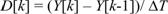(1)

where

D[k] is the drift computed at the current simulation time index,

Y[k] and Y[k-1] are the path errors computed for the current and previous indices, respectively, and

D T is the time step interval.

Values of these variables computed for a given time step are used by the perception module for the next time step.

### Perception

#### Purpose

The perception module translates the physical description of the driving environment into estimates of vehicle states, roadway characteristics, and other relevant variables needed by the decision and control modules. At the user's option, this component provides the decision and control modules with estimates of perceptual variables, relevant to the driving task, that are perturbed by a random disturbance (noise) and/or exhibit a consistent error (bias). The imposition of perceptual noise allows the DVM to treat errors in perceiving and reacting to informational quantities relevant to the driving task, and it provides a mechanism for inducing response variability within and across trials. Perceptual variables are enumerated in the section on Computations below.

#### Assumptions and Limitations

Five assumptions underlie computations in the perceptual module:

• The driver's perceptual process provides the driver with noisy estimates of relevant perceptual variables. This assumption is supported by both psychophysical data and by the results of experiments in manual control. The psychophysical data support the notion of perceptual thresholds, or just-noticeable differences, that characterize the uncertainty and variability in judging whether or not a signal is different from zero or other target value. Manual control data, obtained mostly in laboratory settings under constrained conditions, show that a substantial portion of the human's response behavior is related to variability within the human's information-processing system. For mathematical convenience, this response variability is generally modeled as a random noise process associated with perception.
• The driver estimates the speed appropriate to an upcoming curve based on past experience in traversing similar curved segments. This is an opinion of the authors. In terms of the algorithm specified for the model, the consequences of this assumption are identical to an alternative assumption that the driver estimates the curvature and computes the desired speed based on this estimate.
• The driver effectively flattens the curve by beginning the turning maneuver before curve entry and by beginning the straightening-out maneuver before curve exit. Simulator and on-road data support the hypothesis that some drivers adopt this strategy. As noted above, the user has the option to explore this strategy or to explore the hypothesis that drivers always attempt to remain in the center of the lane.
• The error associated with estimating horizontal curvature decreases in proportion to the distance from curve entry. This reflects a broad underlying assumption, supported by psychophysical experimentation, that the ability to resolve a signal improves in direct proportion to increases in its magnitude. The size of the retinal image of the roadway in the vicinity of the upcoming curve varies inversely with distance to the curve.
• The driver uses the rate of expansion of the optical target image to determine appropriate deceleration for stopping. Experimental data have been reported that are consistent with this hypothesis that drivers use variables based on rate of optical expansion in their braking.

#### Computations

Estimates of the following state variables are computed:

• Longitudinal acceleration.
• Lateral acceleration.
• Yaw (turning) acceleration.
• Yaw rate error, defined as the difference between vehicle yaw rate and the effective roadway yaw rate (i.e., velocity times curvature). In order for the driver to anticipate a curve, yaw rate error is computed from the road curvature one preview distance ahead. The preview distance is computed as the velocity times the time constant computed in the path-control module for yaw-rate control.
• Rate of change of the optical target image at a stopping location. This information is used to generate an appropriate braking strategy.
• Vehicle velocity.
• Distance to a curve entry or stopping point.
• Deviation of vehicle position from lane center (path error).
• Rate of change of lane position (drift).
• Appropriate negotiation speeds for the curves ahead.

Whenever the driver updates the estimate of a particular variable, the new estimate consists of the true simulated variable potentially corrupted by both a bias factor and additive zero-mean Gaussian noise. The bias factor is intended to account for a consistent over- or underestimation of the variable, e.g., a tendency to underestimate vehicle speed. For example, a bias of 0.9 represents a 10 percent underestimation of the magnitude of the variable, 1.1 represents a 10 percent overestimation, and 1.0 represents the lack of a consistent directional error.

Potential sources of estimation bias include underestimation of vehicle velocity as perceived from the visual flow field by driver used to a lower-profile vehicle having a lower eye height, or the misestimation of the horizontal curvature ahead when the curve and approach tangents are on different grades. We are not aware of data to support specific numeric values for nonunity bias factors, however.

In general, a Gaussian random noise process is added to the perceived variable to account for both the effects of perceptual resolution limitations (e.g., thresholds) and for uncertainties that tend to scale with the magnitude of the variable, which are accommodated in the DVM by noise scale factors. Noise processes are modeled as a Gaussian white noise shaped by a first-order filter that limits rates at which instantaneous estimation errors can change over time.

Figure 2 is a flow diagram of the computational procedure. The algorithms for performing the computations are as follows:

Consider the generic variable x that is to be estimated by the driver. Computation of its perceptual estimate involves the following parameters that are assumed constant for the duration of the model run:

T simulation time step (seconds),

x_tc time constant for the noise filter associated with x (seconds),

x_bias bias factor for estimating x (dimensionless),

x_thresh perceptual threshold for x as a noise standard deviation (units of x), and

x_sf scale factor associated with noise component that scales with x (dimensionless).

Also computed in the course of these is the constant:

x_decay filter decay factor associated with estimation error (dimensionless)

where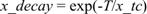. (2)

Finally, the following are recomputed at every time step: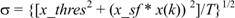(3)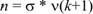(4)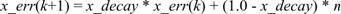(5)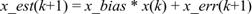(6)

where

k sample index, where each increment corresponds to an advance of T seconds,

x physical variable of interest,

x_est driver's estimate of x,

x_err noise-related estimation error associated with x,

n unfiltered noise sample,

s instantaneous standard deviation associated with n, and

n sample from a unit normal distribution.

Because the driver's ability to estimate the appropriate negotiation speed is assumed to increase with decreasing distance to the curve, the corresponding noise scale factor is computed in the DVM as a curve-noise constant multiplied by the distance to the curve.

A random number generator, modified to approximate a normal probability density, is used to produce each noise sample required by the DVM. Trial-to-trial response variability is obtained by initiating the random number generator with different seeds on successive model runs.

Specifying independent values of filter time constant, bias, threshold, and noise scale factor for every perceptual variable used by the driver would lead to more parameters than experimentally quantifiable. Accordingly, nonzero or otherwise off-nominal values for such variables are selected only where they are expected to materially influence model predictions.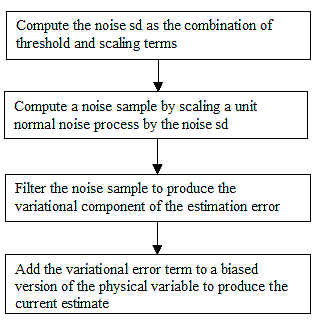sd = Standard deviation

Figure 2. Flow diagram of the computation of perceptual estimates.

The following approach to selecting independent driver-related model parameters is based partly on previous studies of human perception and on model sensitivity analysis. It is consistent with the DVM's primary goal of exploring the effects of highway geometry on speed behavior.

• A single time constant for noise filtering, set at 2 seconds, is applied to all noise processes.
• Noise scale factors, thresholds, and biases are adjusted independently for estimation of current vehicle velocity and for curve negotiation velocity. The scale factors and thresholds have been determined on the basis of realistic driving tasks and/or laboratory psychophysical experiments. The bias terms may be determined from new or published data or may serve as user-adjusted independent parameters of the model analysis.
• A single generic noise scale factor is assigned to all lateral-axis perceptual variables and with longitudinal acceleration. Lateral-axis variables are path error, drift, yaw-rate error, and yaw acceleration.
• Thresholds associated with perception of path error and yaw-rate error are kept as independent parameters and adjusted on the basis of new or published performance data only when more accurate predictions of lateral-axis behavior are desired.
• Thresholds are ignored and bias terms are set to 1.0 in the default driver configurations.

### Path Decision

#### Purpose

The Path Decision component computes the desired track to be followed by the Path Control component.

#### Assumptions and Limitations

One of two possible assumed strategies is followed as determined by the driver type selected by the user:

• Driver attempts to maintain lane center over the entire roadway.
• Driver attempts to cut the curve; i.e., follow a curved path that is flatter than the path defined by the lane center, in the vicinity of a horizontal curve. Otherwise, the driver attempts to track lane center.

#### Computations

An exaggerated diagram of curve cutting is illustrated in figure 3. The actual path of the highway, geometric curve, is indicated by the solid line; whereas the virtual curve path assumed to be followed by the driver is dashed. The virtual curve is a circular arc having the largest radius that begins and ends on lane center, is tangent to the lane edge at these locations, and achieves maximum allowable deviation from the geometric lane center at the midpoint of the geometric curve. The entry points of the geometric and virtual curves are respectively denoted by Sce and Scev.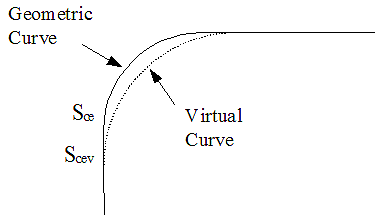Figure 3. Diagram of the assumed path through a horizontal curve.

The virtual radius of curvature Rv and virtual curve entry location Scev may be easily derived under simplifying assumptions. Equations 7 and 8 respectively define: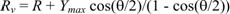(7)

and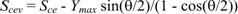(8)

under assumptions of a simple curve of radius R, total deflection angle (i.e., arc length) q , and maximum allowable deviation from center of Ymax. (Ymax is computed as half of the difference between lane width and vehicle width minus the lane margin-a driver parameter reflecting the allowable distance of the vehicle (tractor), e.g., from the lane edge). Note that changes in curve radius and entry depend only on the total curve deflection and maximum lane deviation and not on the geometric radius. If the calculated value for Ymax becomes negative, the DVM terminates with an appropriate end message.

In order for the driver model to exhibit the desired curve-cutting behavior, it is necessary to approximate the path defined above by an analytic expression for which path, drift, and curvature are self-consistent. The following combination of second- and third-order polynomials has been found to provide the desired approximation:

• A second-order polynomial extending from the entry station of the virtual curve to the entry station of the geometric curve.

Parameters are selected such that the curvature of this parabolic segment is approximately that of the curvature of the virtual curve.

• A third-order polynomial from the entry of the geometric curve to the midpoint of the curve.

Parameters are selected such that (a) the displacement and slope of this segment matches that of the parabolic segment at the point of geometric curve entry, (b) the curve attains maximum desired displacement at the curve midpoint, and (c) the curve has zero slope at the curve midpoint. Slope is defined here as the derivative of the path deviation with respect to station.

• A third-order curve that is the mirror image of the foregoing, reflected about the curve midpoint, extending from curve midpoint to the exit station of the geometric curve.
• A parabolic segment that is the mirror image of the initial parabolic segment from the exit station of the geometric curve to the exit station of the virtual curve.

The algorithm for forcing the driver to cut the corner consists of two parts: (1) a set of computations that is completed once per curve, and (2) additional computations that are performed every simulation update.

#### Computations Once Per Curve

The computations given here are performed the first time a horizontal curve becomes relevant and are not performed again until another curve becomes relevant. A curve becomes relevant when it is the next curve ahead once the driver has finished negotiating a curve. The initial curve of the roadway is relevant when the simulation starts. (If the driver is assumed to cut corners, the simulation should begin on a tangent sufficiently ahead of the first curve so that the driver is not immediately turning.)

The following variables serve as inputs to the algorithm:

sce location (station) of the geometric curve entry (m),

scev location of the virtual curve entry (m),

scx location of the geometric curve exit (m),

scxv location of the virtual curve exit (m),

r geometric curve radius (m) (always positive),

ymax maximum deviation from lane center (m) (always positive), and

sgn 1.0 for right curve, -1.0 for left curve.

The following computations are performed once per curve: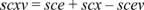(9)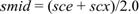(10)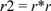(11)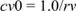(12)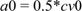(13)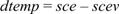(14)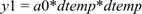(15)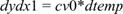(16)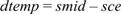(17)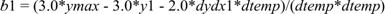(18)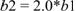(19)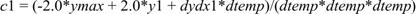(20)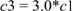(21)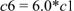(22)

All of these computed variables, with the exception of dtemp, are needed for the updates performed at every simulation interval.

#### Computations Performed Every Simulation Interval

In addition to the inputs and computed variables listed above, the following variables serve as inputs to the algorithm executed every time step:

s current station (m)

v current velocity (m/s)

c curvature of the geometric road at the preview distance (rad/m) (positive for right curve, negative for left)

Note that c is the instantaneous curvature at the preview distance, which is zero if on the tangent, 1/r (with proper sign) if on the geometric curve, and something in between if on a spiral segment.

The following are the primary outputs of this algorithm:

ycorr correction to path error (m),

dcorr correction to drift (m/s), and

yrcorr correction to yaw rate error (rad/sec).

These variables are used to correct the driver's estimates so that the driver attempts to minimize deviations about these desired values rather than about zero. For example: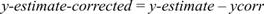(23)

where

y-estimate-corrected estimated path error operated on by the driver, and

y-estimate estimated deviation of lane position from lane center.

The computations described in figure 4 are performed every simulation interval: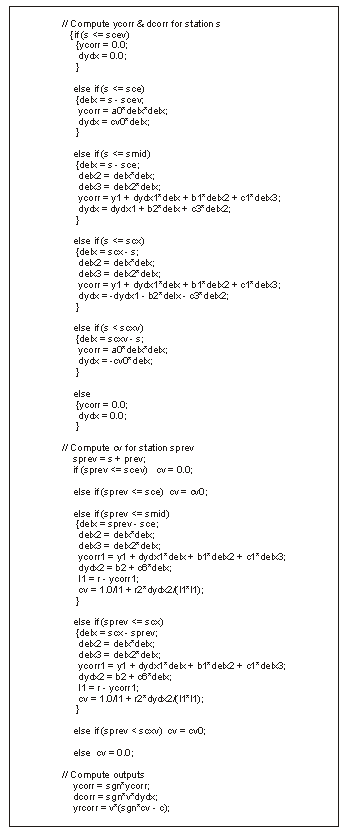Figure 4. Pseudo-code for calculating path decision.

### Path Control

#### Purpose

The Path Control component generates wheel movements to regulate lateral placement (path) by following commands generated by the path decision component.

#### Assumptions and Limitations

• The driver mentally constructs a linear model of the path-regulation task which includes a second-order model of vehicle yaw response.

Data obtained from simulator and on-road studies of driving behavior were consistent with the hypothesis that the driver constructs an adequate mental model of system dynamics. Analysis of the VDM supports a second-order linear approximation to yaw rate response.

• The driver adopts a linear response strategy to control heading and lateral path.

A considerable body of literature supports the assumption that linear models provide good replications of human operator response strategies in many situations where the system to be controlled can be adequately represented as a linearly responding system. Our on-road research results also were consistent with this linear assumption.

#### Computations

A linear strategy is employed using a successive loop-closure technique described below, with the exception that an acceptable tolerance may be specified below which the driver does not attempt to reduce path error. (The tolerance is zero in the default driver configurations provided in the IHSDM.)

This strategy subdivides the control task into a series of subtasks, where, at each step, the system to be controlled is approximated by the combination of an effective time delay t , an integration, and a scale factor Kv. Such a system can be well controlled with a simple feedback gain of: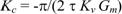(24)

where

the minus sign provides the necessary negative feedback to reduce system errors,

Gm is the gain margin constant,

t is in seconds,

the units of Kv depend on the variable being controlled and the effective control input,

Kc has units that are the reciprocal of t Kv, and

Gm is dimensionless.

Theoretically, the minimum Gm value is 1.0, which represents a controlled system on the verge of instability. Increasing values of Gm provide increasing margins of stability at a cost of increasingly sluggish response. For this driver model, a gain margin of about 3.0 was found to provide a relatively rapid response with little oscillatory behavior.

Figure 5 diagrams an approximation to the task of regulating vehicle lane position, which is modeled as a succession of three subtasks: regulation of yaw-rate error, drift, and path error. Sufficiently small values are assumed for the angle between the vehicle velocity vector and local tangent to the road, the drift rate, and path deviation from lane center such that the sine of any angle variable appearing in the calculations may be replaced by the value of the angle in radians, and its cosine may approximated as unity.

The driver's primary input is considered to be a commanded rate of change of the steering wheel angle. A single integration yields wheel angle, which is related to yaw rate (vehicle rate of turn) by the vehicle dynamics. The yaw-rate error is defined as the difference between the vehicle turn rate (more precisely, rate of vehicle velocity vector rotation) and the effective roadway turn rate (i.e., vehicle velocity times local road curvature). Integration of yaw-rate error yields heading error, i.e., difference between velocity vector and local road tangent. Heading error multiplied by velocity yields a close approximation to drift. Integration of drift subsequently yields path error. The approximation to the steering task shown above is used only for determining the driver's control strategy. Actual vehicle response dynamics are simulated to a high degree of fidelity in the VDM component of the DVM.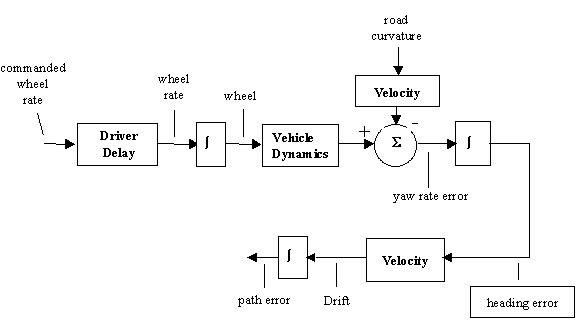Figure 5. Flow diagram of an approximation to the path-regulation task.

For the purpose of computing control gains, vehicle steering dynamics are represented by a second-order filter having a steady state gain Kv relating yaw-rate to steering wheel angle and a natural frequency of w o. Because these parameters are speed dependent, the path control module contains a table of Kv and w o obtained from the yaw-rate response of the VDM at selected speeds. Interpolation of model table entries is performed at each simulation iteration to determine the instantaneous values of Kv and w o

Computation of driver control gains is performed inside out; i.e., the gain related to yaw rate regulation is computed first, then the gain related to drift regulation, and finally the gain related to path regulation. The phase shift of this filter is approximated by an equivalent delay of 0.7/ w o. To compute the gain on yaw-rate error, the driver delay t d is combined with the equivalent vehicle delay to yield an effective delay: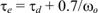(25)

which with application of equation 24 yields the feedback gain Kr on yaw rate error: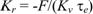(26)

where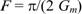(27)

The inner-loop control task of regulating yaw rate is now approximated by a new effective delay of (0.7 t e )/F, where the factor 0.7 matches the phase lags of the yaw-rate control loop and the effective pure delay at about -50 degrees.

The feedback gain on drift is computed by considering the task of controlling drift with a yaw-rate command, where the effective controlled element is now the effective delay mentioned above, plus an integration scaled by velocity. Application of equation 24 to this control task yields the following feedback gain Kd on drift: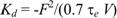(28)

Carrying this process one more step yields the following feedback control gain Ky on path error: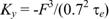(29)

To represent the driver's tolerance of small path errors, the feedback term Ky is set to zero whenever the magnitude of the path error is less than a user-specified tolerance. For improved response, an additional feedback gain of Kr/ w o is applied to yaw acceleration.

To determine the control strategy, the above gains are applied in an outside-in manner. The gain Ky is applied to path error to generate a drift command. The difference between the instantaneous drift and the commanded drift is scaled by the gain Kd to generate a yaw rate command. The commanded wheel rate is computed by adding two terms: (a) the gain Kr acting on the difference between the instantaneous and commanded yaw rates and (b) the gain on yaw acceleration acting on yaw acceleration.

The steering control laws are thus generated in a relatively straightforward manner on the basis of vehicle response parameters and two driver-related parameters: (a) time delay, which is assumed to relate to inherent information-processing capability, and (b) the gain margin, which is assumed to be largely under the driver's control and is considered an aspect of driver preference or driving style.

### Speed Decision

#### Purpose

The speed decision module generates speed or acceleration commands to be acted upon by the speed control module.

##### Assumptions and Limitations

It is assumed that the driver:

• Attempts to maintain a preferred free speed (Vfree) when not limited by highway geometry, posted speed limits, stop signs, or other vehicles. (Other vehicles are not considered in this implementation.)
• Prefers to decelerate at a relatively low constant (preferred) deceleration Axnom. If necessary, a larger (maximum) deceleration not exceeding Axmax will be commanded.
• Estimates the appropriate speed for negotiating a curve ahead on the bases of past experience with curves in general.
• Attempts to negotiate a horizontal curve with a lateral acceleration that varies as the square root of the curvature.

#### Computations

Each time the speed decision module is entered, the situation is analyzed to determine whether the driver needs to decelerate or accelerate. Factors requiring deceleration are:

1. Going faster than desired in a curve.
2. A traffic control device ahead requiring a full stop.
3. A speed advisory for the driver to slow down.
4. A curve ahead that requires the driver to slow down.

If any of these factors requires a deceleration greater than some threshold value, a deceleration is commanded which, if held constant, is expected to bring the car to a stop or to the desired reduced speed as the target is reached. If no deceleration is required, and the vehicle is proceeding at less than the currently desired speed, a command to maintain the currently desired speed is generated.

Prior to the start of the simulation, the DVM computes a desired speed Vc and desired lateral acceleration Ay for each horizontal curve as follows: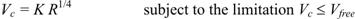(30)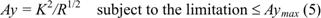(31)

where

K is a constant reflecting vehicle response characteristics as well as driver preferences and is based on both theoretical(1) and empirical(2-4) studies,

Aymax is the maximum tolerable lateral acceleration, and

R is the geometric radius if the driver attempts to maintain lane center or the virtual radius if the driver cuts the curve.

Figure 6 shows the major computational operations of the speed decision module. If the vehicle is negotiating a curve at a speed with excess lateral acceleration, a deceleration of Axmax is commanded. Otherwise, acceleration commands are computed for the following contingencies, where the relevant events are either visible or otherwise known to the driver:

• Driver is assumed to obey posted speed limits (a parameter set by the user),whereby a corresponding acceleration is computed: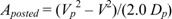(32)

where

Vp = next posted speed,

V = estimated current vehicle speed, and

Dp = estimated distance to the next speed limit sign.

• Next posted speed is less than the posted speed currently operative, whereby a deceleration is computed that would be expected to achieve the next posted speed at the point where indicated (an assumption of the model developers). If the next posted speed limit is higher than the current limit, the acceleration is set to the preferred acceleration.
• Stop sign ahead, whereby the following deceleration is computed which, if held constant, brings the vehicle to a stop at the stop sign location: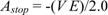(33)

where

E is the fractional rate of expansion of the retinal image formed by the stop sign (or other object at same distance).

The optical expansion (rad/s) approximation used in the DVM for this psychophysical variable is V/Ds, where Ds is distance to the stop sign.

• One or more curves ahead, whereby the following acceleration value is computed which, if held constant, is expected to achieve the desired curve speed at entry: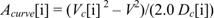(34)

where

Vc = estimated curve negotiation speed as given in equation 30, and

Dc = estimated distance to curve entry.

The largest (negative) acceleration computed for the set of curves is selected as the relevant curve deceleration.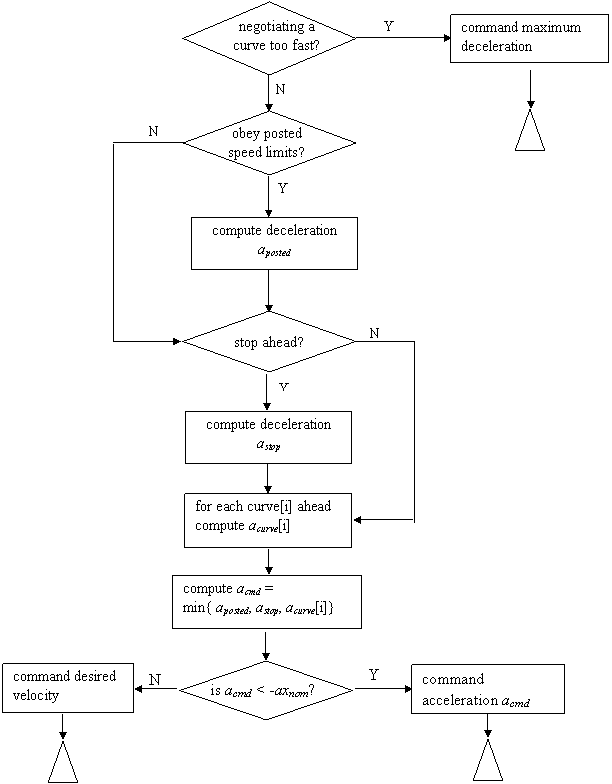Figure 6. Simplified flow diagram of the speed decision logic.

The DVM selects, from the above alternatives, the largest deceleration (i.e., most negative acceleration) as the tentative acceleration command Acmd. If Acmd is less than -Axnom, it is sent to the speed control module as an acceleration command, subject to the restriction of not being more negative than-Axmax. Otherwise, a command is sent to maintain or acquire a velocity that is the minimum of:

• Preferred free speed,
• Current posted speed, if obeying posted speed limit, or
• Appropriate speed for the horizontal curve currently being negotiated.

The speed decision module has additional features. Specifically, if the vehicle has stopped at a stop sign, a counter is set. The decision logic described above is then bypassed until a wait time has passed (user defined). In addition, one of the following treatments is employed for events that are beyond the available SD, depending on the Road Familiarity option set by the user in the IHSDM Administration Tool:

• The driver is familiar with the road and knows what lies beyond. In effect, the SD limitation is ignored.
• The driver assumes a long tangent just beyond the visual range. In effect, nothing lies beyond visual range requiring driver to slow down.
• A maximally cautious driver assumes a stopping requirement just beyond visual range.
• The driver assumes road geometry beyond the SD is similar to that recently negotiated. In effect, a horizontal curve of the same geometry as the curve most recently negotiated is assumed.

The long-tangent assumption has been selected for the default driver configurations provided in the IHSDM.

Highway-related factors influencing SD in the DVM are vertical crest and obstruction offsets. The driver model also contains a variable representing the driver's maximum SD. This variable, accessible through the Administration Tool, is set to 1,000 m in the default driver configurations. A lower value could be selected to reflect environmental (e.g., weather) limits to SD.

#### More accurately model the relation between acceleration and required speed change:

The DVM assumes that the driver's preferred deceleration or acceleration during curve approach and after curve exit is the same for all horizontal curves. The data, however, are more consistent with the assumption that such accelerations and deceleration vary with the square root of the total desired speed change. A theoretical construct has been defined that predicts the fore-mentioned square-root relationship between the acceleration or deceleration and the desired speed change.(3) Existing data obtained in the on-road studies could be used to quantify the parameters of this model.

In order for such a model to be applicable, however, it is necessary to have a model for predicting the desired tangent speeds between two curves. We originally suggested that an optimization algorithm be sought to develop such a model-an effort that we consider to be beyond the scope of the present effort.

At the suggestion of the FHWA, we agreed to explore the approach taken in a previous study.(5) This study was not entirely applicable, however, as it explored only long tangents, defined as tangents where the driver can reach and maintain the desired speed. Furthermore, the authors concluded that their findings "indicated that combinations of alignment indices and other geometric variables were not able to significantly predict the 85th percentile speeds of motorists on long tangents of two-lane rural highways."

Two other studies of speed on two-lane rural highways were reviewed. Figueroa and Tarko(6) developed regression models for predicting mean speed and speed dispersions in curves and on tangent approaches to curves. They developed a 12-factor model for mean speed, but curvature was characterized simply by a binary value (1 if radius greater than 1,700 ft, 0 otherwise), and tangent length and characteristics of preceding curve were not considered.

Polus, Fitzpatrick, and Fambro(1) explored and developed regression models for the effects of preceding and following horizontal curves on the intervening tangent speeds. They concluded that tangent length and radii of preceding and following curves were the most important variables in the regression equations. They also concluded that a single model for tangent speed was inadequate because of the low R2, and they subsequently developed four models using descriptors of the highway environment based on curve radii and intervening tangent length. While this study is the most relevant of the three studies reviewed here, we have decided not to attempt to implement the results in the DVM for the following reasons:

1. The models are limited to pairs of preceding and following horizontal radii that are either both less than or equal to 250 m, or both greater than 250 m.
2. Insufficient data exist to validate one of the models.
3. The model forms are markedly different from the component models currently used in the DVM, and it is not clear that sufficient resources are available for implementation and testing of these models in the current study.

We suggest that an optimization approach similar to that used in the current DVM be pursued in a subsequent model development effort.

### Speed Control

#### Purpose

The speed control component generates accelerator and brake pedal actions to regulate the speed or acceleration as commanded by the speed decision component.

#### Assumptions and Limitations

The accelerator and brake are operated by a single foot which is always in one of the three following conditions:

1. Operating the accelerator,
2. Operating the brake, or
3. Transitioning between brake and accelerator.

The driver adopts a linear control strategy to regulate either speed or acceleration.

#### Computations

The speed control algorithm consists of: (1) a wait period, in which no action is taken, to reflect the time it takes the driver to transition from one pedal to the other; (2) a time delay to reflect delays associated with the driver's perceptual/motor response, and (3) three bounded linear systems. The latter convert a speed-command into an acceleration command, operate the accelerator to achieve the desired acceleration, and operate the brake to achieve the desired (negative) acceleration.

Pedal movements are for automatic transmission vehicles. The driver model does not handle gear shifting.

In general, the speed decision model issues an acceleration command when the vehicle is required to slow down or stop for an event ahead and otherwise issues a velocity command.

When a velocity command Vcmd is received from the speed decision module, the following commanded acceleration Acmd is computed: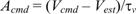(35)

where

Vest is the estimated vehicle velocity,

t v is a velocity time constant, and

|Acmd| is limited to Axnom.

The acceleration command-either computed as above or provided directly by the speed decision module-is then filtered as follows to provide a commanded rate of change of the pedal Prate: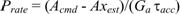if the accelerator pedal is being operated, or (36)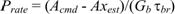if the brake pedal is being operated (37)

where

Axest is the estimated longitudinal acceleration,

Ga is the accelerator pedal gain,

t acc is a time constant associated with the accelerator pedal, and

Gb and t br are similar parameters associated with operation of the brake.

The accelerator and brake gains are constants approximating the change in speed (m/s) per unit of pedal deflection, where 1.0 represents a maximum deflection. The time constants are selected to provide a subjectively satisfactory system response (i.e., timely but minimally oscillatory). The magnitude of the commanded rate of pedal movement is restricted to a maximum value specified by the user (e.g., maximum rate of full deflection in 1/2 second used in DVM testing to date).

The resulting command is delayed by the assumed driver delay (typically, 0.2 s) to yield a delayed pedal rate. The accelerator pedal displacement is incremented during the simulation update interval according to this pedal rate if the accelerator pedal is currently being controlled. Brake pedal displacement is otherwise incremented.

A pedal transition is initiated whenever:

• A negative pedal rate is commanded signifying further deceleration.
• The brake pedal is being controlled, the pedal is at zero displacement, and a positive pedal rate is commanded signifying further acceleration.

We suggest that an optimization approach similar to that used in the current DVM be pursued in a subsequent model development effort. This assumption represents a worst-case driver behavior and thus provides the most critical test of the highway design from a safety standpoint.

With transition, a counter is incremented according to the pedal transition time (user specified). When the speed control module is re-entered, the count is decremented, and the module is exited if the count has not reached zero. Control is switched to the other pedal after transitioning is completed.

Speed and throttle must be initialized to reflect the vehicle state at the start of the simulation. In particular, the influence of posted speed, nearby curves and stop signs, and the possibility of starting in a curve must be considered.

The following variables are defined:

axnom = nominal acceleration

ay = lateral acceleration

aymax = maximum allowable lateral acceleration

Vfree = driver's preferred (free) speed

V = temporary speed variable

Vc = curve negotiation speed

Vdes = desired initial speed

R = effective curve radius (positive number)

D = temporary distance variable

The pseudo-code in figure 7 demonstrates the logic to be implemented.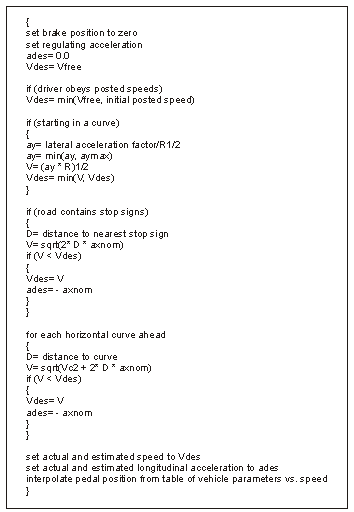Figure 7. Pseudo-code for calculating speed control.

## Vehicle Dynamics Model (VDM)

The VDM included in the DVM is based on the vehicle dynamics analysis, non linear (VDANL) model, which is intended for the analysis of passenger cars, light trucks, articulated vehicles, and multi-purpose vehicles. At present only the passenger car and Class 8 tractor trailer are simulated in the DVM.

A detailed description of the VDM is beyond the scope of this document. A brief review of the principal features of this model is given here, abstracted from material provided by the VDANL developer. The reader is referred to Allen et al.(2) for additional details.

Model equations cover the full range of lateral/directional and longitudinal motions up through large angles experienced in spin out and rollover. The vehicle model includes components for sprung and unsprung masses, suspension, steering, braking, power train, drive train, and tires. The model includes a comprehensive tire model and properly accounts for the effects of maneuver-induced load transfer. The vehicle and tire models are based on past research and have been extensively validated.

Major elements of the VDM include:

• Tires: Characteristics of these play a dramatic role in vehicle dynamics since they respond to vehicle maneuvering. The tire model generates lateral and longitudinal tire forces and aligning moments as functions of normal load, slip, and camber angle and includes appropriate interactions between these input variables including force saturation.
• Suspension: Composite suspension characteristics are designed to represent wheel steer and camber motions relative to the sprung mass and squat/lift forces resulting from tire ground plane forces acting on the suspension geometry. Wheel steer also arises from compliance in response to tire side force and aligning torque.
• Steering: This model includes Ackerman steer effects and compliance and a composite second order characteristic to simulate steering dynamics in response to steering and aligning torque inputs.
• Power and Drive Train: This model includes engine, transmission, differentials and torque splitting between the front and rear axles. Front, rear, and four-wheel-drive can be accommodated. (Defaults are front wheel drive for the passenger car and both drive axles on the truck tractor.)
• Brakes: This model includes simulation of vacuum boost run-out and a nonlinear proportioning valve between the front and rear axles. Also includes a generic anti-lock brake system. Truck brakes include trailer pneumatic lag and fade due to overheating.

Vehicle characteristics are specified in a set of text files that are provided with the IHSDM software.

Outputs of the VDM include the location of the vehicle in geographic coordinates, vehicle heading, path (i.e., lateral offset from lane center), and other vehicle state variables (e.g., yaw rate and longitudinal and lateral accelerations).

## General Specifications

The following are additional specifications that do not specifically correspond to any of the above components.

### Halt Simulation upon Vehicle Rollover

The DVM currently computes the lateral load transfer at each simulation interval. The simulation should halt with an appropriate error message whenever this variable is equal to or greater than 1.0 or equal to or less than -1.0.

The Battelle Team conducted a mini user requirements analysis (URA) in an attempt to identify an appropriate definition for the off-road condition. Representative end-users of the DVM software were asked to provide the Battelle Team with both definitions for and operational uses of the term "off-road" condition. The results from those participating in this analysis were varied, ranging from defining off-road as the inside of a tire being outside the paved section of roadway to the entire vehicle being outside the paved roadway.

The intent of defining an off-road condition in the DVM is both to identify a situation where the driver can be considered to have lost effective control of the vehicle and define a condition where the validity of small-signal linearization adopted in certain computations is in question. (Small-signal approximations should be valid as long as the vehicle remains relatively close to lane center.)

Accordingly, an off-road condition should be defined as a condition in which all tires are entirely off the paved section of roadway (including paved shoulders). When such a condition occurs, the simulation should be halted with an appropriate message. In the case of a Class 8 truck, an off-road condition is to be identified if all tractor wheels are outside the paved section.

If an off-road condition is identified, the simulation is halted with an appropriate message shown to the user.

### Treatment of Curves that are Close Together

The vehicle tends to run off the road when reverse curves are too close together and the driver is trying to cut the curve, especially when the entry point of the second virtual curve is reached before the exit point of the first virtual curve.

Tests were performed using various combinations of horizontal curvatures and separations between successive curves in an attempt to find ways to modify the degree of curve cutting (and therefore the location of the virtual curve entry and exit points) to avoid predicted steering problems. No such modification scheme was found that would consistently produce reasonable steering behavior for close curves. It was noted, however, that reasonable steering behavior was predicted when curves were separated by at least 10 m.

The recommended solution to this problem, if there is at least one pair of curves separated by less than 10 m, is to provide a message to the user at the beginning of the simulation listing each pair of curves separated by less than 10 m, and allow the user the option of continuing or halting the simulation. For simulations involving multiple trials, this message and option should be presented only at the beginning of the first trial.

## Specification for Modifying the Developmental DVM

Two modifications of the DVM have been required to facilitate calibration of the heavy vehicle: (1) additions to the output data file and (2) capability for user-programmed controls.

### Additions to the Output Data File

In order to show the path followed by the heavy vehicle trailer in a horizontal curve, the DVM also includes positions of the outside trailer wheels in the primary output data file (e.g., a file having a name like "analysis.slowtruk.i.csv").

The output data file has been expanded to include the stationing and lateral location of eight wheel positions. The corresponding user-friendly names for the wheel variables (contained in the third row of the data file) should be s-wheel0, s-wheel1, etc., for stationing and y-wheel0, y-wheel1, etc., for lateral position The program names for these variables (contained in the second row of the data file) are at the discretion of the program developer. Lateral positions are given as the distance from lane center in the expanded output data file.

### User-Programmed Controls

The capability for the user to override the driver's control response in the DVM with a pre-programmed control response is required for calibration driver parameters when a new vehicle is to be explored. A developmental version of the DVM was suggested in which the user may independently specify the control authority (driver or model user) for wheel, pedal, and brake. The control profile specified by the user consists of one or more segments of constant control in which each segment is specified in terms of duration and control value. Because vehicle response is time based, time rather than station serves as the independent variable.

After the user requests the simulation to commence, the developmental DVM reads a comma-separated file, UserDriverControls.txt, with the following format:

wheelController, pedalController, brakeController

wheel, pedal, brake, startTime, stopTime

...

wheel, pedal, brake, startTime, stopTime

where

String wheelController = {"User," "Driver"}

String pedalController = {"User," "Driver"}

String brakeController = {"User," "Driver"}

double wheel

double pedal

double brake

double startTime

double stopTime

If the first line of the file contains the string Driver,Driver,Driver, the remainder of the file is ignored, and the DVM proceeds as normal, where all controls are calculated by the driver model. If one or more of the strings in the first line is User, the second line of the file is read, and the values corresponding to all controls designated as user override the values computed by the driver model. Values associated with a driver control do not override the DVM-computed values. The program terminates with an appropriate error message if the value for startTime is not zero, or if the value for stopTime is less than the start time.

If the file contains only two lines of text, the final value of the second line serves as the stop time for the simulation. If the file contains a third line, control and timing variables are read. The simulation halts with an error message if either the start time is not identical to the previous stop time or the stop time is less than the start time. This procedure is repeated until there are no further lines of text in the input file, in which case the final stop time serves as the stopping time for the simulation.

If the simulation is run with partial or full User control, the simulation halts with an error message if the end of the road is reached before the current stop time.

Internally, the procedure for using these control data is as follows:

Store these data in an internal lookup table. Right before calling VDANL, refer to the lookup table to see what values should be used at the current time. For each variable being controlled by User, override the calculated values with the file values; otherwise use the values calculated by the driver model.

## DVM Parameters that are Selectable

The default setting of driver-related parameters in the DVM is intended to represent the behaviors of drivers who are attentive, well-trained, and driving under normal nonemergency conditions on two-lane rural highways in the absence of other traffic. Accordingly, the model generates predictions of acceptable vehicle control for even severe highway geometrics. The DVM does not treat turning at intersections.

The one exception to this rule is the long-tangent treatment of SD limitations discussed previously, but this could be modified to reflect more conservative driving behavior. By modifying certain driver-related parameters, however, one can explore degradations in driving performance resulting from one or more types of information-processing impairment.

The following discussion is organized as follows: (1) Options Selectable by the User, describing the default operation of the DVM, and (2) Options Selectable by the System Administrator, in which manipulations of certain independent model parameters can be made with the existing DVM implementation to explore nonoptimal driver behavior.

### Options Selectable by the User

The DVM allows the user to select among sixteen sets of driver configurations which specify the vehicle being driven (passenger car or tractor-trailer), the nature of the simulation (deterministic or stochastic), and certain driver behaviors. Table 2 lists the sixteen standard configurations provided in the DVM. The user may specify additional configurations via the Administration Tool (also listed as Appendix A).

Table 2. Standard driver configurations.
Configuration Vehicle Driver Type Cuts Curve?
Deterministic nominal-center/Taurus Passenger car Nominal N
Deterministic nominal-cutcurve/Taurus Passenger car Nominal Y
Deterministic aggressive-center/Taurus Passenger car Aggressive N
Deterministic aggressive-cutcurve/Taurus Passenger car Aggressive Y
Deterministic nominal-center/truck Truck Nominal N
Deterministic nominal-cutcurve/truck Truck Nominal Y
Deterministic aggressive-center/truck Truck Aggressive N
Deterministic aggressive-cutcurve/truck Truck Aggressive Y
Stochastic nominal-center/Taurus Passenger car Nominal N
Stochastic nominal-cutcurve/Taurus Passenger car Nominal Y
Stochastic aggressive-center/Taurus Passenger car Aggressive N
Stochastic aggressive-cutcurve/Taurus Passenger car Aggressive Y
Stochastic nominal-center/truck Truck Nominal N
Stochastic nominal-cutcurve/truck Truck Nominal Y
Stochastic aggressive-center/truck Truck Aggressive N
Stochastic aggressive-cutcurve/truck Truck Aggressive Y

where:

"Nominal" approximates the average response characteristics of the test drivers.

"Aggressive" approximates the 85th percentile driver.

Not cutting the curve means the driver attempts to maintain lane center in horizontal curves.

Cutting the curve means the driver tracks to the inside of horizontal curves.

Deterministic configurations assume the absence of variability in driver perception and response. Repeated simulations of a given driving condition will give repeatable results.

Stochastic configurations account for perceptual and response variability as a set of noise processes acting on selected perceptual response variables. A random number generator drives the various perceptual noise processes. A series of model runs with the seed of the random number generator changed from run to run will generate a series of driver responses that allow the user to compute statistics on predicted driver and vehicle response.

Driver characteristics consist of (1) nominal-center, (2) nominal-cutcurve, (3) aggressive-center, and (4) aggressive-cutcurve. The parameters for the nominal-center car driver were obtained by matching the average behavior of 18 car drivers participating in the previous DVM study. The driver is assumed to attempt to maintain the vehicle in the center of the lane over the entire roadway. The nominal-cutcurve driver has the same parameter values as the nominal-center except that the driver is assumed to cut horizontal curves by tracking toward the inside of the curve to lessen the sharpness of the path traveled and thereby allow a higher safe speed when negotiating the curve. Lane-center position is maintained on tangent segments.

The aggressive-center and aggressive-cutcurve drivers differ from their respective nominal drivers in terms of three parameters that have been readjusted to represent the estimated 85th-percentile driver in terms of free speed, lateral acceleration factor, and preferred longitudinal acceleration. Changes in these parameters allow the aggressive driver to cruise at a higher speed in the absence of highway geometric factors (recall that there are assumed to be no other vehicles on the road), take curves at higher speeds and tolerate greater lateral accelerations, and brake more aggressively.

Values for independent model parameters were calibrated on the basis of simulated and on-road studies of passenger cars. The primary goal of the recent DVM study was to adjust the driver parameters as needed to account for the behavior of truck drivers.

The maximum SD for these configurations was assumed to be 1,000 m. An SD this large will typically have no influence on driver behavior. The instantaneous SD, however, may be reduced during a simulation run by highway geometric factors such as sharp vertical crests. In the standard configurations the driver assumes that a long tangent lies beyond the instantaneous SD. In other words, the driver ignores all potential perils that are unseen. One consequence of this treatment may be to cause the driver to react later to a horizontal curve and brake more severely than would be the case with adequate SD.

For these standard configurations, the instantaneous desired speed is the minimum of (1) the free speed, (2) the speed allowed by a requirement to stop ahead, and (3) the speed dictated by one or more curves ahead.

The procedure for calibrating driver parameters is discussed in Section 4.

### Options Selectable by the System Administrator

#### Speed limits

The standard options assume that drivers ignore speed limits. The user can specify a new Driver in which posted speed limits are assumed to be obeyed. In this case, the desired speed will never be greater than the prevailing speed limit (which can be different for different segments of the highway). It may be less if the driver's desired free speed is less than the posted speed.

As noted earlier, the DVM allows for four alternative treatments of SD limitations. The standard configurations reflect the driver's assumption that a long tangent lies just beyond the visual range; i.e., that there are no events requiring the driver to slow down. Other treatments may be employed by defining new driver configurations.

#### Maximum Sight Distance

Additional Drivers can be defined with lesser value of maximum SD to reflect visibility limitations caused by weather conditions. Reducing the maximum SD may also be implemented to impose a situation in which the driver does not respond to a horizontal curve in a timely manner due to inattention or aggressiveness.

#### Maximum Allowable Lateral Acceleration

We assume that truck drivers will adopt maximum lateral accelerations that are less than the rollover threshold; i.e., the lateral acceleration that would cause the truck to tip over. The ratio of maximum allowable acceleration to rollover threshold provides a measure of rollover stability in a turn. On-road studies indicate that the relative stability is not constant, but varies with the loading on the truck. Specifically, drivers appear to tolerate a larger lateral acceleration relative to rollover threshold (and therefore greater risk of rollover) for loaded trucks compared to unloaded trucks.